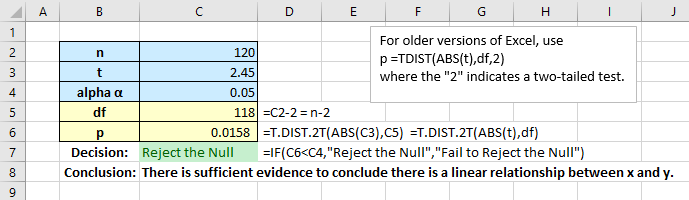# Simple Regression Part 3

When you are given raw data on a test, solving the problem using technology is relatively simple. But when you are given intermediates or partial solutions, the task becomes a bit more difficult since most software is not set up to work on intermediates.

Consider the following problem:

A scientist employed simple linear regression to model the monthly price of recycled newspaper as a function of the monthly price of pulpwood. The results shown below were obtained for monthly data collected over a recent  10-year period (n = 120 months).

Use this information to conduct a simple linear regression analysis:

ŷ = 35.20+5.28x; for testing H0: β1 = 0, t = 2.45; r = 0.22; r2 = 0.05.

Solution: What is meant by “conduct a simple linear regression analysis”? We are given the regression equation, r and r2, so that part is already done. The clue is we are given a t-value for “testing H0: β1 = 0.” This indicates we need to conduct a hypothesis test of the slope to confirm it is non-zero and the model is useful.

The alternative hypothesis for these tests is Ha: β1 ≠ 0, the complement of the null. Thus, this is a two-tailed test.

First, using Excel:The StatCrunch approach: Use the command sequence Stat > Calculators > T. Enter the degrees of freedom, 118, the t of 2.45, select the right tail, and click Compute. Because this is a two-tail test, multiply the indicated p-value of 0.00788 by 2 to get p = 0.0158. Again, we decide to reject the null and conclude there is sufficient evidence of a linear relationship between x and y.